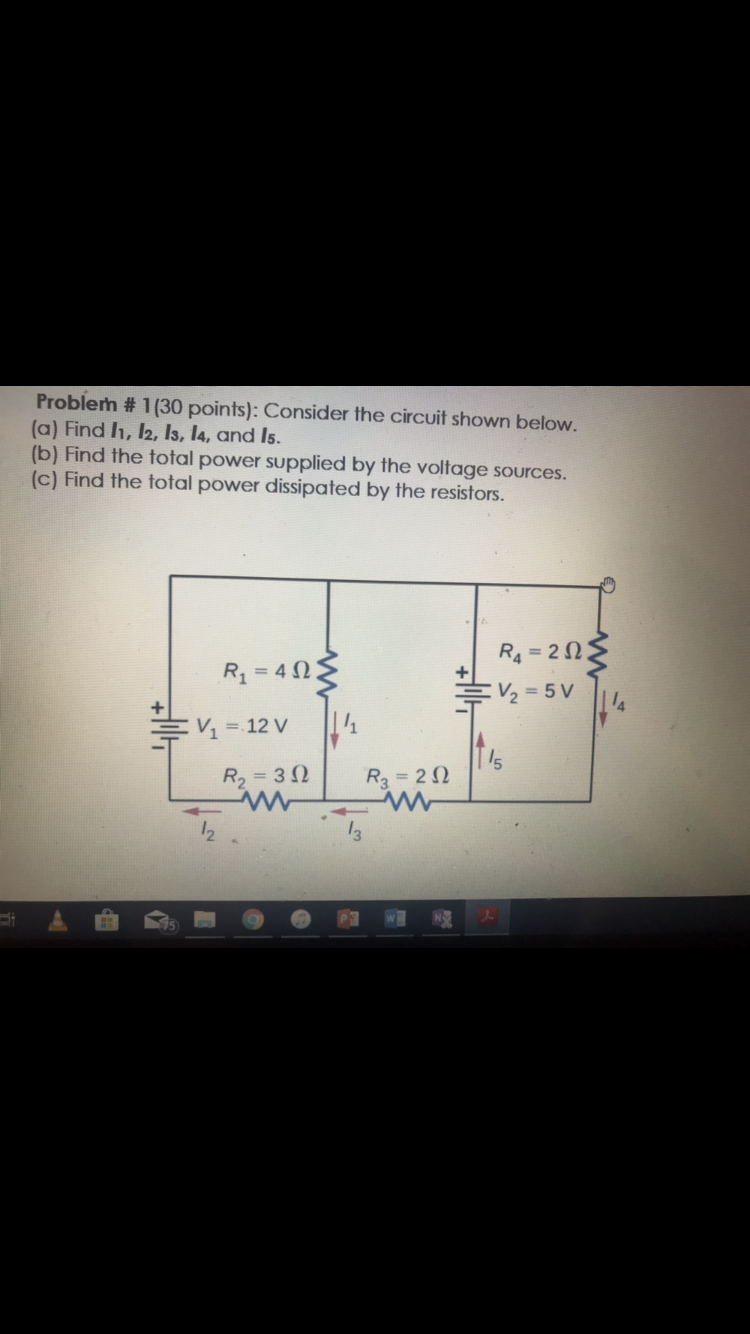# Problem # 1 (30 points): Consider the circuit shown below. (a) Find h, 12, Is, l4, and Is. (b) Find the total power supplied by the voltage sources. (c) Find the total power dissipated by the resistors. R4 -20 V 12V R2 3R3 2 2 3

Questionhelp_outlineImage TranscriptioncloseProblem # 1 (30 points): Consider the circuit shown below. (a) Find h, 12, Is, l4, and Is. (b) Find the total power supplied by the voltage sources. (c) Find the total power dissipated by the resistors. R4 -20 V 12V R2 3R3 2 2 3 fullscreen

### Want to see the step-by-step answer?